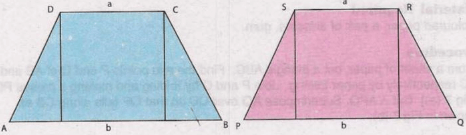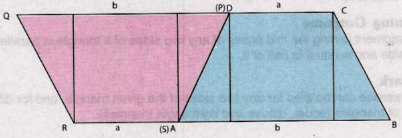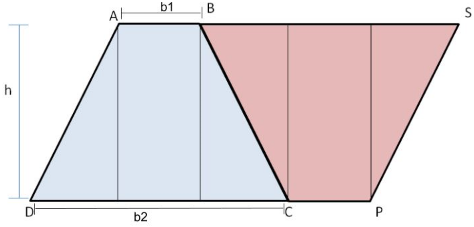# Procedure - Area of Trapezium is Equal to half the Product of its Altitude, Class 9 Math Class 9 Notes | EduRev

## Class 9 : Procedure - Area of Trapezium is Equal to half the Product of its Altitude, Class 9 Math Class 9 Notes | EduRev

The document Procedure - Area of Trapezium is Equal to half the Product of its Altitude, Class 9 Math Class 9 Notes | EduRev is a part of the Class 9 Course Mathematics (Maths) Class 9.
All you need of Class 9 at this link: Class 9

As performed in the real lab:

Materials Required:

Coloured paper, a pair of scissors, gum.

Procedure:

1. Take two sheets of coloured paper.

2. Cut two identical trapeziums ABCD and PQRS as shown in figure.

3. Paste them together along PS with AD.

4. It will forms parallelogram.

5. Calculate area of trapezium.As performed in the simulator:

1. Create a Trapezium ABCD by providing length of 2 bases (parallel sides) and height

2. Don't give both base value same otherwise it will form rectangle rather than Trapezium.

3. Click on a Trapezium ABCD to create a Trapezium PQRS

4. Click twice on PQRS to rotate PQRS in 180 degree.

5. Drag trapezium PQRS and place at appropriate position such that it will forms a parallelogram.

6. Place trapezium PQRS such that leg BC of trapezium ABCD will touch leg RQ of trapezium PQR.Observation:

1.Parallelogram ASPD is formed by combining two trapezium ABCD And BSPC.

2.Area of Trapezium ABCD = 1/2 X Area of parallelogram DASP
=1/2 X (DC+CP) X h
=1/2 X (b1 + b2) X h

Result:

The area of trapezium is half the product of its altitude and sum of its parallel sides.

Offer running on EduRev: Apply code STAYHOME200 to get INR 200 off on our premium plan EduRev Infinity!

## Mathematics (Maths) Class 9

190 videos|233 docs|82 tests

,

,

,

,

,

,

,

,

,

,

,

,

,

,

,

,

,

,

,

,

,

,

,

,

;